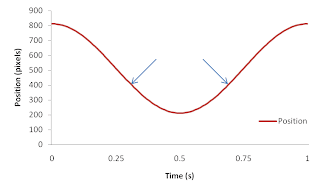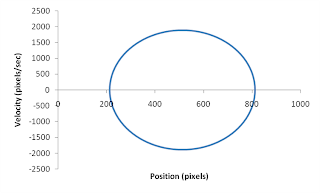Tuesday, 14 December 2010

How to Build a Valid Measure of Behaviour

One of the main problems facing psychology as a science is the issue of validity - what is the relationship between what you measured and what you are actually interested in? One of the things I like about studying movement is how straight-forward this issue is - we're interested in the control of action, so I just measure the action! The most common directly measured kinematic variable is displacement, or position over time; you can then derive (via differentiation) the various rates of change of the previous variable (velocity, acceleration, jerk, and, I kid you not, snap, crackle, and pop). In human movement we never tend to go past jerk, and you can do pretty well with just position and it's rate of change, velocity.

This post will discuss how we start from these basic kinematics and derive a measure of coordination that is  entirely valid, covers the entire space of possible states and provides a unique number for every possible state within that space. Psychology doesn't have a lot of these kinds of variables, but you need to be able to characterise your state space to do the kind of modelling I've been describing and advocating.

If I want to characterise a rhythmic movement (and I often do) I begin by directly measuring a time series of position for each oscillator. Let's take just one, which typically looks like this:Figure 1. Position time series of a 1Hz oscillator from one of my experimental displays
Position, however, isn't that useful a dependant variable for characterising a rhythmic movement: as you can see from the arrows, the same position occurs twice within a cycle; there is a many-to-one mapping between the variable position and behaviour.

These two positions are distinguished from each other by their direction; the first time it reaches that point the oscillator is heading towards peak amplitude, the second time it's heading away from there. Direction is a component of the vector quantity velocity (the magnitude of the vector is the speed): if we differentiate the position signal we get a velocity time series, and every position/velocity combination is now unique:Figure 2. Velocity times series for the data from Figure 1 (which is shown for reference)
But we now have a problem: we need two numbers to characterise behaviour at each instant of time, and we need one for analysis purposes. The two numbers can't simply be multiplied together, because they are expressed in different units (pixels, and pixels/sec); the resulting number would be in pixels^2/sec which doesn't mean anything obvious. A mathematically legitimate way to combine two numbers from different scales is to plot them, one against each other. The resulting graph of position x velocity is known as a phase portrait, which means this is a figure of the possible states of a system:Figure 3. Unscaled phase portrait of the rhythmic oscillator
We still need a single number to identify each unique (position, velocity) coordinate; the fact that the resulting plot is a circle suggests we might want to use an angle, which will range from 0° to 360° around that circle and thus provide a single number for each coordinate. That angle is formed by a line from the centre of the plot to the (position, velocity) combination at each moment in time, and can be computed by applying simple trigonometry to the resulting triangle.

We first make the problem simpler by normalising the data so as to centre the data on (0,0) and make the plot a unit circle (radius = 1). This doesn't change the form of the plot, but it equates the scale of the axes so when I compute the phase angle the computation is legitimate.

The algorithm is simply
1. Subtract the mean position from the position time series to centre it on zero.
2. Compute velocity
3. Divide the position time series by peak amplitude; position is now a number that ranges from -1 to +1.
4. Divide the velocity by the angular frequency; velocity now also ranges between -1 and +1.Figure 4. Computation of a phase angle (click for bigger)
The trigonometry is now trival: θ is equal to the inverse tangent (arctangent) of the velocity (the 'opposite' side) over the position (the 'adjacent' side). Every possible state in a cycle of a rhythmic movement now has a unique number assigned to it, and thus, given the number, we know the current state of the system.

I now have a measure, phase, of 'position within a cycle'. It satisfies all my requirements (every possible state has a unique value, i.e. there is a 1:1 mapping of behaviour to the value dependant variable; it is perfectly valid, etc).

Coordination
I'm not studying rhythmic movements, though: I'm studying coordinated rhythmic movements. I can compute a phase angle for each of the two oscillators at any given moment in time, but I'm back in the position of needing two numbers to describe each state. Combining these is more straight forward, as they are both expressed in the same units (degrees or radians); what I'm interested in is how different these angles are at each moment in time, so I can simply take the difference between two phase angles to regain a single number which fully characterises the system and preserves all the benefits: relative phase.

Summary
Why am I spending time on this? Several reasons:

First, it will come in handy later when I review and try to rebut a recent paper on how the Watts governor is actually representational, and also when I talk about the problems that arise when you try to analyse relative phase using conventional statistical methods.

Second, I think it's vitally important to be able to know precisely where your variables come from and why you use them, I wrote the analysis code I still use when I was a PhD student, and I hardly ever look at it any more. It's good for me to keep this process in mind to help keep my eye on the ball. The dynamic pattern approach made the critical error of making relative phase the order parameter, and thus assuming that relative phase, per se, is the thing being controlled by the perception/action system. This led to all their predictions, most of which have simply failed to hold up.

It's important to keep in mind that relative phase is a 'world' variable; it's a description of the coordination, the thing to be specified, and not necessarily the information doing the specifying. If the Bingham model is right (and the evidence so far is good) then the information for relative phase is actually the relative direction of motion, and it's with respect to this that behaviour is organised. This three part division - world, perception and perceiver - is the ecological scheme laid out in Turvey, Shaw, Reed and Mace (1981) which Ken's been blogging about and I've mentioned before, and this will come up when I get, finally, to reviewing Tony's book. There's a clarity to this phase analysis that I hope will be as useful to others as it is to me; another reason coordinated rhythmic movement is such a useful model system.

Plus I love the process of building a valid measure of the behaviour you're interested in. It's an exciting part of being a scientist, coming up with ways to answer the question at hand, and this was how I learned the process.

1 comment:

1.Nice article! Quick question.

Suppose the position variable of the raw signal was composed of two axes (or more - coordinations don't happen along one axis) might the same signal processing technique outlined above be implemented? Or would the addition of a second position axis at the raw signal fundamentally break the "phase" approach?

If you can't answer it that's cool! You might be able to answer if having a 2nd position axis is even all that important to your approach. If so, why not?